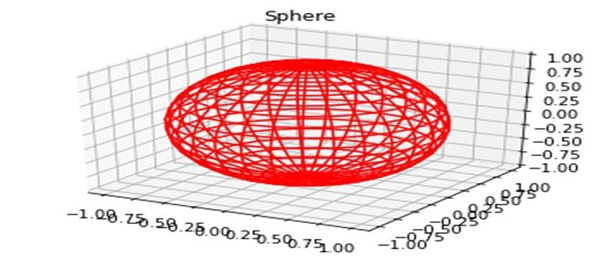# Make 3D plot interactive in Jupyter Notebook (Python & Matplotlib)

In this article, we can take a program code to show how we can make a 3D plot interactive using Jupyter Notebook.

## Steps

• Create a new figure, or activate an existing figure.

• Create fig and ax variables using subplots method, where default nrows and ncols are 1, projection=’3d”.

• Get x, y and z using np.cos and np.sin function.

• Plot the 3D wireframe, using x, y, z and color="red".

• Set a title to the current axis.

• To show the figure, use plt.show() method.

## Example

import matplotlib.pyplot as plt
import numpy as np
fig = plt.figure()
plt.show()